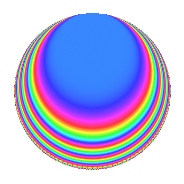Properties

 Label 3600.1.bh.bLevel 3600 Weight 1 Character orbit 3600.bh Analytic conductor 1.797 Analytic rank 0 Dimension 4 Projective image $$D_{6}$$ CM disc. -3 Inner twists 8

Related objects

Newspace parameters

 Level: $$N$$ = $$3600 = 2^{4} \cdot 3^{2} \cdot 5^{2}$$ Weight: $$k$$ = $$1$$ Character orbit: $$[\chi]$$ = 3600.bh (of order $$4$$ and degree $$2$$)

Newform invariants

 Self dual: No Analytic conductor: $$1.79663404548$$ Analytic rank: $$0$$ Dimension: $$4$$ Relative dimension: $$2$$ over $$\Q(i)$$ Coefficient field: $$\Q(i, \sqrt{6})$$ Coefficient ring: $$\Z[a_1, \ldots, a_{19}]$$ Coefficient ring index: $$1$$ Projective image $$D_{6}$$ Projective field Galois closure of 6.2.450000.1

$q$-expansion

Coefficients of the $$q$$-expansion are expressed in terms of a basis $$1,\beta_1,\beta_2,\beta_3$$ for the coefficient ring described below. We also show the integral $$q$$-expansion of the trace form.

 $$f(q)$$ $$=$$ $$q$$ $$-\beta_{1} q^{7}$$ $$+O(q^{10})$$ $$q$$ $$-\beta_{1} q^{7}$$ $$+ \beta_{3} q^{13}$$ $$+ \beta_{2} q^{19}$$ $$- q^{31}$$ $$+ \beta_{3} q^{43}$$ $$+ 2 \beta_{2} q^{49}$$ $$- q^{61}$$ $$+ \beta_{1} q^{67}$$ $$+ 2 \beta_{2} q^{79}$$ $$+ 3 q^{91}$$ $$-\beta_{1} q^{97}$$ $$+O(q^{100})$$ $$\operatorname{Tr}(f)(q)$$ $$=$$ $$4q$$ $$\mathstrut +\mathstrut O(q^{10})$$ $$4q$$ $$\mathstrut -\mathstrut 4q^{31}$$ $$\mathstrut -\mathstrut 4q^{61}$$ $$\mathstrut +\mathstrut 12q^{91}$$ $$\mathstrut +\mathstrut O(q^{100})$$

Basis of coefficient ring in terms of a root $$\nu$$ of $$x^{4}\mathstrut +\mathstrut$$ $$9$$:

 $$\beta_{0}$$ $$=$$ $$1$$ $$\beta_{1}$$ $$=$$ $$\nu$$ $$\beta_{2}$$ $$=$$ $$\nu^{2}$$$$/3$$ $$\beta_{3}$$ $$=$$ $$\nu^{3}$$$$/3$$
 $$1$$ $$=$$ $$\beta_0$$ $$\nu$$ $$=$$ $$\beta_{1}$$ $$\nu^{2}$$ $$=$$ $$3$$ $$\beta_{2}$$ $$\nu^{3}$$ $$=$$ $$3$$ $$\beta_{3}$$

Character Values

We give the values of $$\chi$$ on generators for $$\left(\mathbb{Z}/3600\mathbb{Z}\right)^\times$$.

 $$n$$ $$577$$ $$901$$ $$2801$$ $$3151$$ $$\chi(n)$$ $$\beta_{2}$$ $$1$$ $$1$$ $$1$$

Embeddings

For each embedding $$\iota_m$$ of the coefficient field, the values $$\iota_m(a_n)$$ are shown below.

For more information on an embedded modular form you can click on its label.

Label $$\iota_m(\nu)$$ $$a_{2}$$ $$a_{3}$$ $$a_{4}$$ $$a_{5}$$ $$a_{6}$$ $$a_{7}$$ $$a_{8}$$ $$a_{9}$$ $$a_{10}$$
2593.1
 1.22474 − 1.22474i −1.22474 + 1.22474i 1.22474 + 1.22474i −1.22474 − 1.22474i
0 0 0 0 0 −1.22474 + 1.22474i 0 0 0
2593.2 0 0 0 0 0 1.22474 1.22474i 0 0 0
3457.1 0 0 0 0 0 −1.22474 1.22474i 0 0 0
3457.2 0 0 0 0 0 1.22474 + 1.22474i 0 0 0
 $$n$$: e.g. 2-40 or 990-1000 Significant digits: Format: Complex embeddings Normalized embeddings Satake parameters Satake angles

Inner twists

Char. orbit Parity Mult. Self Twist Proved
1.a Even 1 trivial yes
3.b Odd 1 CM by $$\Q(\sqrt{-3})$$ yes
5.b Even 1 yes
5.c Odd 2 yes
15.d Odd 1 yes
15.e Even 2 yes

Hecke kernels

This newform can be constructed as the kernel of the linear operator $$T_{7}^{4}$$ $$\mathstrut +\mathstrut 9$$ acting on $$S_{1}^{\mathrm{new}}(3600, [\chi])$$.